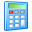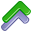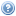Widgets# Bending Springback Calculator

After a bending operation, residual stresses will cause the sheet metal to spring back slightly. Due to this elastic recovery, it is necessary to over-bend the sheet a precise amount to acheive the desired bend radius and bend angle. The final bend radius and bend angle can be approximated from the sheet thickness, K-factor, material yield strength and modulus of elasticity, and the initial bend angle and bend radius. The final bend radius will be greater than initially formed and the final bend angle will be smaller. The springback factor, Ks, will show the ratio of the final bend angle to the initial bend angle. Learn more about Bending.

## Bending Springback Calculator

Sheet thickness (in):